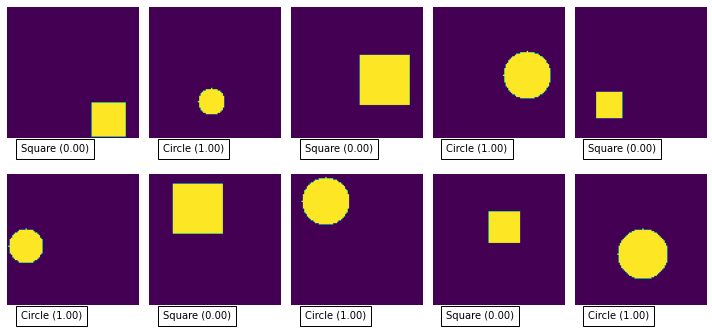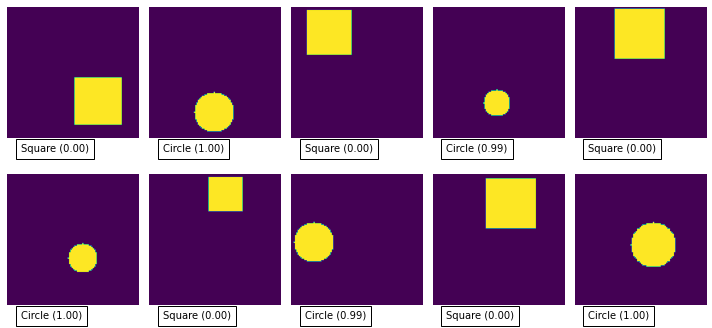Article Image
Article Image

Binary classification is used where you have data that falls into two possible classes - a classic example would be “hotdog” or “not hotdog” ((if you don’t get the hot dog reference then watch this).

If you’re looking to categorise your input into more than 2 categories then checkout TensorFlow Categorical Classification

You can find the example notebook(s) for this post in the tensorflow-tutorial GitHub repo.

For this small tutorial, I’ve created a python generator that creates images with either a square or a circle.def data_generator():
i = 0
while(True):
if i >= 1000:
i = 0
# our output value will be 0 or 1
Y = i % 2
X = np.zeros((image_width, image_height, 1))

# size of our shape
# position of our shape

if Y == 0: # generate a square
else: # generate a circle
X[y+center_y, x+center_x] = 1
yield X, [Y]
i = i + 1


Our simple generator will generate an infinite number of samples, alternating between a random square and random circle.

To get binary classification working we need to take note of a couple of things:

1. We need to have one output neuron with a sigmoid activation function. The sigmoid activation function will return a value between 0 and 1 - we’ll use this to determine how confident the network is that input falls the true class.
2. We need to use the BinaryCrossentropy loss function during our training.

Our simple model looks like this:

model = Sequential([
Conv2D(8, 3,
activation='relu',
input_shape=(image_width, image_height, 1),
name='conv_layer'),
MaxPooling2D(name='max_pooling'),
Flatten(),
Dense(
10,
activation='relu',
name='hidden_layer'
),
Dense(1, activation='sigmoid', name='output')
])


And when we compile it we specify the loss function that we want to optimise:

model.compile(optimizer='adam',
loss=tf.keras.losses.BinaryCrossentropy(),
metrics=['accuracy'])


Given our simple problem (is it a square or a triangle) you should be able to get close to 100% accuracy with just a few training epochs.

You can test the model pretty easily by feeding in some more random samples from the training set:

# get a batch of samples from the dataset
X, Y = next(iter(train_dataset))
# ask the model to predict the output for our samples
predicted_Y = model.predict(X.numpy())
# show the images along with the predicted value
plot_images(X, predicted_Y)As you can see it is pretty good at classifying the images, mostly producing 0 or 1 for each image.

Checkout the full code in the GitHub repo.

> Image